# Python | 变量类型详解

Python 中的变量赋值不需要类型声明。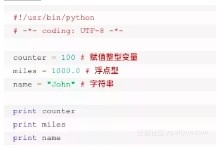1001000.0John

Python允许你同时为多个变量赋值。

a = b = c = 1

a, b, c = 1, 2, "john"

Python 定义了一些标准类型，用于存储各种类型的数据。

Python有五个标准的数据类型：

Numbers（数字）

String（字符串）

List（列表）

Tuple（元组）

Dictionary（字典）

Python数字

var1 = 1

var2 = 10

del语句的语法是：

del var1[,var2[,var3[....,varN]]]]

del var

del var_a, var_b

Python支持四种不同的数字类型：

int（有符号整型）

long（长整型[也可以代表八进制和十六进制]）

float（浮点型）

complex（复数）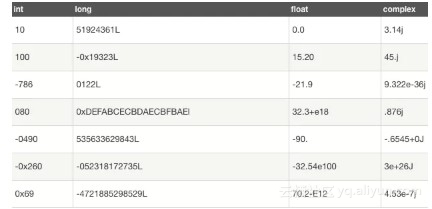Python还支持复数，复数由实数部分和虚数部分构成，可以用a + bj,或者complex(a,b)表示， 复数的实部a和虚部b都是浮点型

Python字符串

s="a1a2···an"(n>=0)

python的字串列表有2种取值顺序:

s = 'ilovepython'

s[1:5]的结果是love。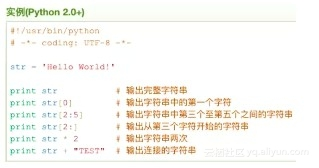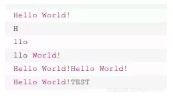Python列表

List（列表） 是 Python 中使用最频繁的数据类型。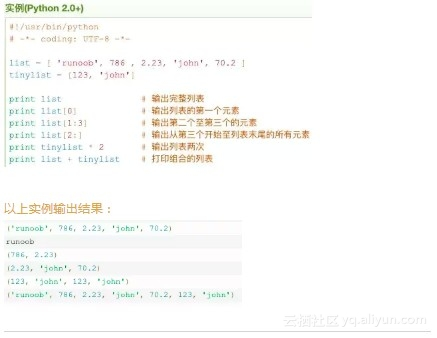Python元组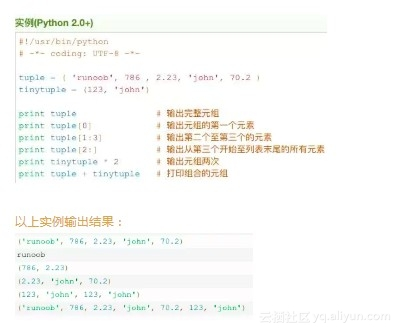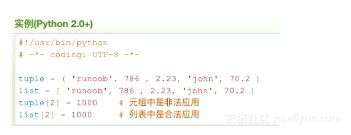Python 字典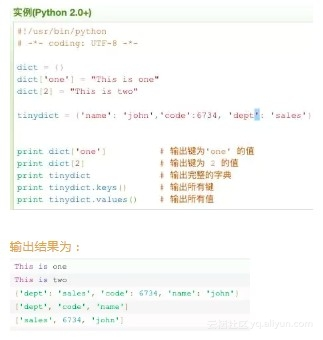Python数据类型转换

int(x [,base])

long(x [,base] )

float(x)

complex(real [,imag])

str(x)

repr(x)

eval(str)

tuple(s)

list(s)

set(s)

dict(d)

frozenset(s)

chr(x)

unichr(x)

ord(x)

hex(x)

oct(x)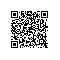使用钉钉扫一扫加入圈子
+ 订阅